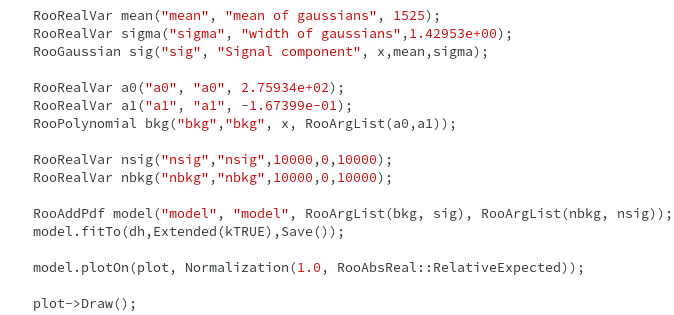# Extended Likelihood

Hi everyone!

I am trying to extract upper limits on Number of signal events. I have a Gaussian signal over flat background. My question is if I fit my data with model:

``````               Model = Nsig * gauss + Nbkg * poly
``````

And use option i.e. Extended(kTRUE) as shown in the code bellow. Does this mean it automatically fits like:

``````              Nsig/(Nsig+Nbkg) * gauss + Nbkg/(Nsig+Nbkg) * poly
``````

with Poisson term multiplied by it?And what happened if i don’t use “Extended” option with it?

Another question is why we add constraint term with our model. Is in my case, this Poisson term is called Constraint term?

Hi @cosmogenic!

Yes, it’s true that if you create a `RooAddPdf` where the coefficients represent the number of events (i.e. they don’t add up to one), RooFit will do an extended fit and give you fit results for `nsig` and `nbkg` with uncertainties. The `Extended` option will be ignored here, so if you don’t use it you will still get an extended fit because of the way you defined the `model`.

If you don’t want to do an extended fit, you have to define the model with `n-1` coefficients in the range [0,1]:

``````   RooRealVar frac("frac","background fraction", 0.5, 0., 1.);
``````

I don’t completely understand your question about the constraint term. Yes, the Poisson term from the extended fit is a constraint term, because it is a constraint on a global observable, in this case the number of events.

And just a little suggestion: if you want to easily see the fit results without the fitting log, you can silence the fit prints and pretty-print the fit result only:

``````RooFitResult * result = model.fitTo(dh, Save(true), PrintLevel(-1));
result->Print();
delete result;
``````

I hope this answer helps you, if you have any further questions please let me know!

Cheers,
Jonas

Dear @jonas

Thank you very very much. That helps alot!
One more question, may be its too basic but i want to clear myself:

What is the difference between Unbinned and Extended Likelihood?

Thank you!

Actually what i wanted to know was that can i put more that one constraints on model (i.e. the model that already contain Poisson constraint term) or not? Like if i define my model as extended model and want to put constraints on some other parameters so can i simply multiply my Extended model with those constraints?

Like in rf604_constraints.C, they are multiplying model with constraint.

Hi @cosmogenic!

In a binned fit, the measurements are first binned in histograms. Then you have a Poisson distribution for the number of events in each bin, with the means being the expected number of events. You evaluate these Poisson probabilities and take the product over all bins for the full likelihood. Because you use the number of expected events in each bin for the model, you are using also the information of the total number of observed events in this way.

In an unbinned fit, the model pdf is evaluated for each each measurement, and the product is the likelihood. Because you only use the differential shape information here (via your pdf) and not the total number of events, you have to add the extra Poisson term to account for this information. That’s the extended term.

So to answer your question: the difference between unbinned and extended likelihood is only the Poisson constraint term for the total number of events.

You can find more information on this on the internet, for example in this presentation.

And yes, you can add further constraints in any way described in the rf604_constraints.C tutorial, even if the likelihood is already an extended likelihood!

I hope things are a bit clearer now, and if not don’t hesitate to ask again!

Cheers,
Jonas

Thank you very very much! Now these concepts are clear to me.

This topic was automatically closed 14 days after the last reply. New replies are no longer allowed.